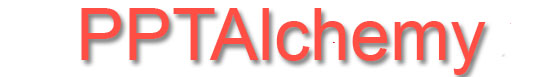PowerPoint tips, hints and tutorials that will change your presentations for ever!

INDEXMock Table Soup

Tables are good for showing information but sometimes they cannot do what you need.

Suppose you want to use each cell as a trigger or animate the cells individually.

To get round this you can make your own mock table by duplicating shapes and carefully arranging them into a mock table! This can be painstaking.

This vba does it for you. Place ONE shape where you want the table to start, select it and run the code. We suggest that the shape you use has an outline of about 2 points.

Sub Mock_Table_Soup()
Dim sRow As String
Dim sCol As String
Dim R As Long
Dim C As Long
Dim sngH As Single
Dim sngW As Single
Dim sngT As Single
Dim sngL As Single
Dim oshp As Shape

'check one shape selected
If ActiveWindow.Selection.Type <> ppSelectionShapes Then GoTo Err
If ActiveWindow.Selection.ShapeRange.Count <> 1 Then GoTo Err
Set oshp = ActiveWindow.Selection.ShapeRange(1)
'get position
With oshp
sngH = .Height
sngW = .Width
sngL = .Left
sngT = .Top
End With

'Get input check numeric
Do
sCol = InputBox("Number of Columns?")
If StrPtr(sCol) = False Then Exit Sub
Loop Until IsNumeric(sCol) And Val(sCol) > 0
Do
sRow = InputBox("Number of Rows?")
If StrPtr(sRow) = False Then Exit Sub
Loop Until IsNumeric(sRow) And Val(sRow) > 0

'make the mock table
For R = 0 To CLng(sRow) - 1
For C = 0 To CLng(sCol) - 1
With oshp.Duplicate
.Left = sngL + (C * sngW)
.Top = sngT + (R * sngH)
End With
Next C
Next R
oshp.Delete
Exit Sub
Err:
MsgBox "Please select ONE shape", vbCritical
End Sub

Now add the main shape positioned exactly below the first header cell. Select it and choose the SAME NUMBER of columns and the desired number of rows.

Neil Humphries saw our code and adapted it for his own needs to report on how many columns and rows could fit on a slide and allow the creation of spaces between the shapes.

Here is Neil's code:

Sub Mock_Table_Soup2()
'Based on Mock_Table_Soup by PPTAlchemy
Dim sRow As String
Dim sCol As String
Dim R As Long
Dim C As Long
Dim sngH As Single
Dim sngW As Single
Dim sngT As Single
Dim sngL As Single
Dim sSH As String
Dim sSW As String
Dim sGH As String
Dim sGV As String
Dim sngMG As Single
Dim oshp As Shape

'check one shape selected
If ActiveWindow.Selection.Type <> ppSelectionShapes Then GoTo Err
If ActiveWindow.Selection.ShapeRange.Count <> 1 Then GoTo Err
'get slide dimensions
sSW = Application.ActivePresentation.PageSetup.SlideWidth
sSH = Application.ActivePresentation.PageSetup.SlideHeight
'Debug.Print "Slide Width " & sSW & " Slide Height " & sSH
'get position
Set oshp = ActiveWindow.Selection.ShapeRange(1)
With oshp
sngH = .Height
sngW = .Width
sngL = .Left
sngT = .Top
End With
'Debug.Print "Shape Width " & sngW & " Shape Height " & sngH

'Get input check numeric
Do
sCol = InputBox("Up to " & Int((sSW - sngL) / sngW) & " columns will fit.", "Number of Columns?")
If StrPtr(sCol) = False Then Exit Sub
Loop Until IsNumeric(sCol) And Val(sCol) > 0

sngMG = (sSW - sngL - (sCol * sngW)) / (sCol - 1)
Do
sGH = InputBox("Maximum gap between columns is " & Round(sngMG, 2) & " points, " & vbCrLf & vbCrLf _
& vbTab & "( " & Round(sngMG / 72, 3) & " inches, " & Round(sngMG / 72 * 25.4, 1) & " mm )", _
"Space between columns in points?")
If StrPtr(sGH) = False Then Exit Sub
Loop Until IsNumeric(sGH)
Do
sRow = InputBox("Up to " & Int((sSH - sngT) / sngH) & " rows will fit.", "Number of Rows?")
If StrPtr(sRow) = False Then Exit Sub
Loop Until IsNumeric(sRow) And Val(sRow) > 0

sngMG = (sSH - sngT - (sRow * sngH)) / (sRow - 1)
Do
sGV = InputBox("Maximum gap between rows is " & Round(sngMG, 2) & " points, " & vbCrLf & vbCrLf _
& vbTab & "( " & Round(sngMG / 72, 3) & " inches, " & Round(sngMG / 72 * 25.4, 1) & " mm )", _
"Space between rows in points?")
If StrPtr(sGV) = False Then Exit Sub
Loop Until IsNumeric(sGV)

'make the mock table
For R = 0 To CLng(sRow) - 1
For C = 0 To CLng(sCol) - 1
With oshp.Duplicate
.Left = sngL + (C * (sngW + sGH))
.Top = sngT + (R * (sngH + sGV))
'Debug.Print .Left & ", " & .Top
End With
Next C
Next R
oshp.Delete
Exit Sub

Err:
MsgBox "Please select ONE shape", vbCritical
End Sub

Don't know how to use vba - see here

Back to the Index Page